## Friday, April 8, 2016

### Average trees & the visualization of tree space using multidimensional scaling

Following up on some earlier posts (1, 2) on averaging the phylogenies in a sample (say, a Bayesian posterior sample), I thought it might be interesting to explore the distribution of the original trees and their average using a multidimensional scaling (MDS) visualization of tree space.

Since treespace is kind of difficult to visualize, one of the approaches that has been proposed (here by Hillis, Heath, & St. John in 2005) is to project the distances between trees into a lower dimensional space, such as 2 or 3D Euclidean space, using the standard statistical technique of MDS.

Here's a quick demo in which I first compute the Robinson-Foulds minimum-distance (average) tree, and then the differences between all trees, including the average tree, then project these distances into 2D space using MDS:

``````library(phytools)
library(phangorn)
trees
``````
``````## 100 phylogenetic trees
``````
``````rf.tree<-averageTree(trees,method="symmetric.difference")
``````
``````##
##   Function is attempting to find the phylogeny with
##   minimum distance to all trees in set under the
##   "symmetric difference" criterion...
``````
``````##   Best SS so far = 18720
``````
``````##   Best SS so far = 18520
``````
``````##   Best SS so far = 16108
``````
``````##   Best SS so far = 15992
``````
``````##   Best SS so far = 15932
``````
``````##   Best SS so far = 15116
``````
``````##   Best SS so far = 14808
``````
``````##   Best SS so far = 13172
``````
``````##   Best SS so far = 12424
``````
``````##   Best SS so far = 11348
##   Best SS so far = 11348
``````
``````##
##   Solution found after 11 set of nearest neighbor interchanges.
``````
``````tmp<-list(rf.tree)
class(tmp)<-"multiPhylo"
obj<-c(trees,tmp)
D<-RF.dist(obj)
MDS<-cmdscale(D)
plot(MDS,xlab="MDS axis 1",ylab="MDS axis 2")
for(i in 1:nrow(MDS))
lines(c(MDS[i,1],MDS[nrow(MDS),1]),c(MDS[i,2],MDS[nrow(MDS),2]),
col="grey")
points(MDS[,1],MDS[,2],pch=21,bg="grey")
points(x=MDS[nrow(MDS),1],y=MDS[nrow(MDS),2],pch=21,bg="red")
abline(v=0,h=0,lty="dotted")
d.mds<-colSums(as.matrix(dist(MDS)))
ii<-which(d.mds==min(d.mds))
points(x=MDS[ii,1],y=MDS[ii,2],pch=21,bg="blue")
``````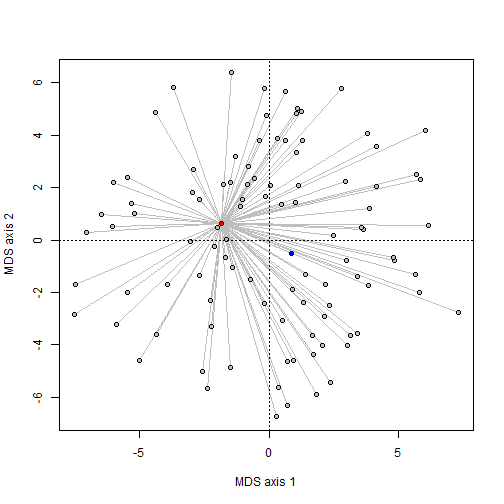Unfortunately, this is not a great example - because although our average tree on the RF criterion does have the lowest distance to the other trees in the set, when projected into the MDS space it doesn't! This is one of the shortcomings of MDS is that distances from high-dimensional data (such as phylogenies) sometimes does not project well into 2D space. For fun, I computed the tree with the lowest distance in MDS space and that point is shown as blue, above.

This tree is not particularly good, which we can see as follows:

``````par(mfrow=c(2,1))
plotTree(compute.brlen(root(obj[[ii]],outgroup=c("Lemur","Tarsier"),
resolve.root=TRUE)),mar=c(0.1,0.1,4.1,0.1))
title(main="Minimum distance tree in MDS space")
plotTree(root(rf.tree,outgroup=c("Lemur","Tarsier"),resolve.root=TRUE),
mar=c(0.1,0.1,4.1,0.1))
title(main="Average tree by RF criterion")
``````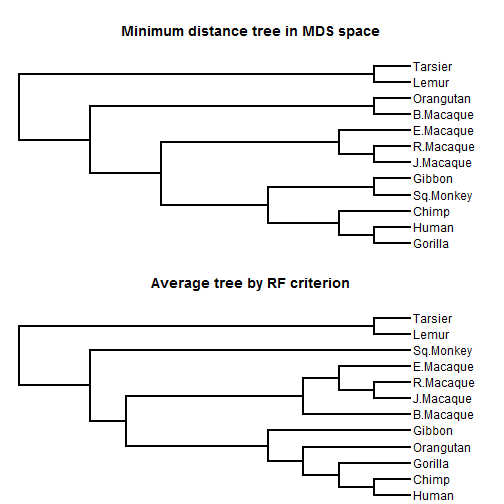What can I say? Anyone can see the first tree is bad.

We can help explain this when we look at how poorly correlated distances in RF space & MDS space are:

``````plot(dist(MDS[1:100,]),as.dist(as.matrix(D)[1:100,1:100]),pch=21,bg="grey",
xlab="distance in MDS space",ylab="distance in original tree space")
points(as.matrix(dist(MDS))[101,1:100],as.matrix(D)[101,1:100],pch=21,
bg="red")
``````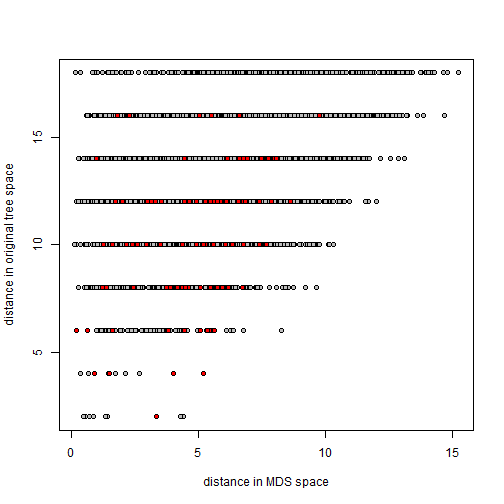The red points show the distances from the average tree.

Though it might be tempting to assume that it does, this minimum distance tree in the MDS space needn't be the median tree - that is, the tree from the set with the minimum distance to the other trees of the set.

We can find this median tree as follows:

``````d<-colSums(as.matrix(RF.dist(trees)))
ii<-which(d==min(d))
par(mfrow=c(2,1))
plotTree(compute.brlen(root(trees[[ii]],outgroup=c("Lemur","Tarsier"),
resolve.root=TRUE)),mar=c(0.1,0.1,4.1,0.1))
title(main="Median tree by RF criterion")
plotTree(root(rf.tree,outgroup=c("Lemur","Tarsier"),resolve.root=TRUE),
mar=c(0.1,0.1,4.1,0.1))
title(main="Average tree by RF criterion")
``````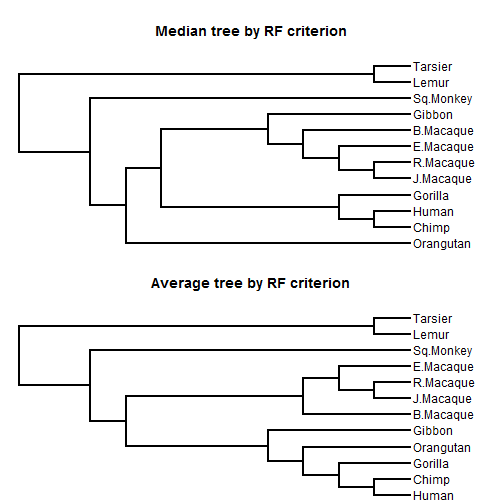(Clearly, the median tree is also not too good!)

Next, I'll repeat the same thing with a distance I haven't talked about yet which I'll refer to as quadratic path difference, and will come up again

``````qpd.tree<-averageTree(trees,method="quadratic.path.difference")
``````
``````##
##   Function is attempting to find the phylogeny with
##   minimum distance to all trees in set under the
``````
``````##   Best SS so far = 75.2236158072111
``````
``````##
##   Solution found after 1 set of nearest neighbor interchanges.
``````
``````tmp<-list(qpd.tree)
class(tmp)<-"multiPhylo"
obj<-c(trees,tmp)
D<-phytools:::qpd(obj,obj)
MDS<-cmdscale(D)
plot(MDS,xlab="MDS axis 1",ylab="MDS axis 2")
for(i in 1:nrow(MDS))
lines(c(MDS[i,1],MDS[nrow(MDS),1]),c(MDS[i,2],MDS[nrow(MDS),2]),
col="grey")
points(MDS[,1],MDS[,2],pch=21,bg="grey")
points(x=MDS[nrow(MDS),1],y=MDS[nrow(MDS),2],pch=21,bg="red")
abline(v=0,h=0,lty="dotted")
``````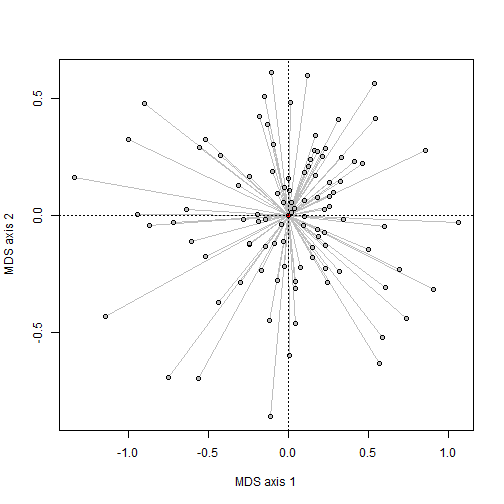Here, it seems quite clear that the MDS does a much better job at representing the distribution of trees, and in fact this is borne out when we compare the distances in MDS and the original space:

``````plot(dist(MDS[1:100,]),as.dist(D[1:100,1:100]),pch=21,bg="grey",
xlab="distance in MDS space",ylab="distance in tree original space")
points(as.matrix(dist(MDS))[101,1:100],D[101,1:100],pch=21,bg="red")
``````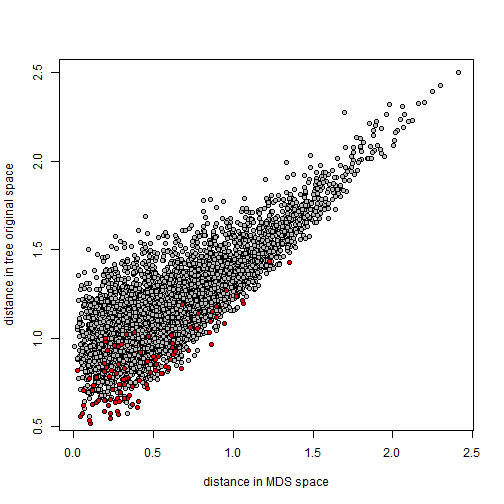At this point I can't really say what any of this means with regard to how we should go about computing the average tree, or what distance metrics are best at representing our data; however hopefully that will come!